Dams valley management¶

Consider two dams in the same valley. These two dams are connected together, as we suppose that the water turbined by the first dam is an input of the second dam. The goal of this notebook is to show how to use the Stochastic Dual Dynamic Programming (SDDP) algorithm to find how to manage optimally these two dams.

Mathematical formulation:¶

Assume that we have a water inflow $W_t$ which arrives in the first dam between $t$ and $t+1$. This dam turbines a quantity $U_t^1$ of water, and spill a quantity $S_t^1$.

Its dynamic is:

$$X_{t+1}^1 = X_{t}^1 + W^t - U_t^1 - S_t^1$$

The turbined flow arrives in the second dam, which turbines a quantity $U_t^2$ and spills a quantity $S_t^2$. So its dynamic is:

$$X_{t+1}^2 = X_{t}^2 + U_t^1 + S_t^1 - U_t^2 - S_t^2$$

Thus, we could define the state: $$X_t = (X_t^1, X_t^2)$$

and the control: $$U_t = (U_t^1, U_t^2, S_t^1, S_t^2)$$

The two turbines produce a quantity of electricity proportionnal to the flow turbined, and this electricity is sold into the market at a price $c_t$. So we gain at each timestep:

$$C_t(X_t, U_t) = c_t (U_t^1 + U_t^2)$$

Here, we suppose that costs are negative, as we sell electricity onto the network.

We want to maximize our expected gains, so we minimize the following quantity:

$$J = \mathbb{E} \left[ \sum_{i=1}^{T_f} C_t(X_t, U_t) \right]$$

Problem formulation:¶

First, we need to import some modules:

In :
using JuMP, Clp, StochDynamicProgramming, PyPlot
INFO: Recompiling stale cache file /home/fpacaud/.julia/lib/v0.5/PyPlot.ji for module PyPlot.

JuMP is the julia module for Mathematical Programming, Clp the module calling a linear solver (can be replaced by CPLEX or Gurobi), StochDynamicProgramming is a SDDP module in Julia, PyPlot is used here to plot the results

Constants definition¶

Then, we define the constants of this problem:

In :
# Number of timesteps (as we manage the dams over a year, it is equal to the number of weeks):
TF = 52

# Capacity of dams:
VOLUME_MAX = 100
VOLUME_MIN = 0

# Specify the maximum flow that could be turnined:
CONTROL_MAX = round(Int, .4/7. * VOLUME_MAX) + 1
CONTROL_MIN = 0

# Some statistics about aleas (water inflow):
W_MAX = round(Int, .5/7. * VOLUME_MAX)
W_MIN = 0
DW = 1

T0 = 1

# Define aleas' space:
N_ALEAS = Int(round(Int, (W_MAX - W_MIN) / DW + 1))
ALEAS = linspace(W_MIN, W_MAX, N_ALEAS);

Now, we generate a random process to simulate the evolution of electricity prices over a year:

In :
COST = -66*2.7*(1 + .5*(rand(TF) - .5));

We could plot the evolution of prices with matplotlib:

In :
plot(COST, color="k", lw=2)
xlim(0, 53)
xlabel("Week")
ylabel("Cost")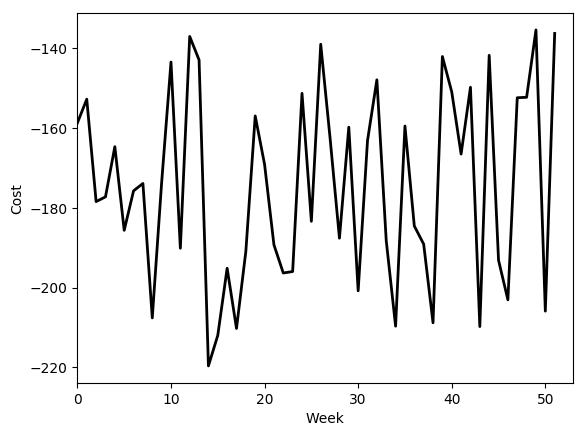Out:
PyObject <matplotlib.text.Text object at 0x7f1aa19ef710>

Dynamic, costs and aleas¶

We could now define the dynamic of our system:

In :
# Define dynamic of the dam:
function dynamic(t, x, u, w)
return [x - u - u + w, x - u - u + u + u]
end
Out:
dynamic (generic function with 1 method)

and the cost at time $t$:

In :
# Define cost corresponding to each timestep:
function cost_t(t, x, u, w)
return COST[t] * (u + u)
end
Out:
cost_t (generic function with 1 method)

Now, we build a function to simulate the evolution of water inflow over one year. We aim to have less water in summer than in winter.

In :
"""Build aleas probabilities for each month."""
function build_aleas()
aleas = zeros(N_ALEAS, TF)

# take into account seasonality effects:
unorm_prob = linspace(1, N_ALEAS, N_ALEAS)
proba1 = unorm_prob / sum(unorm_prob)
proba2 = proba1[N_ALEAS:-1:1]

for t in 1:TF
aleas[:, t] = (1 - sin(pi*t/TF)) * proba1 + sin(pi*t/TF) * proba2
end
return aleas
end

"""Build an admissible scenario for water inflow."""
function build_scenarios(n_scenarios::Int64, probabilities)
scenarios = zeros(n_scenarios, TF)

for scen in 1:n_scenarios
for t in 1:TF
Pcum = cumsum(probabilities[:, t])

n_random = rand()
prob = findfirst(x -> x > n_random, Pcum)
scenarios[scen, t] = prob
end
end
return scenarios
end
Out:
build_scenarios

We could test our generator with one scenario:

In :
scenario = build_scenarios(1, build_aleas());

plot(scenario', lw=2, color="blue")
xlabel("Week")
ylabel("Water inflow")
xlim(0, 53)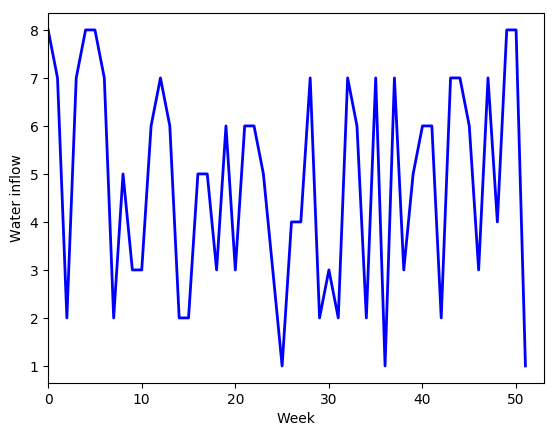Out:
(0,53)

To use these scenarios in SDDP, we must use a discrete distribution for each timestep. The following function generates n_scenarios and returns a vector of NoiseLaw corresponding to the evolution of aleas distribution along the time:

In :
"""Build probability distribution at each timestep.

Return a Vector{NoiseLaw}"""
function generate_probability_laws(n_scenarios)
aleas = build_scenarios(n_scenarios, build_aleas())

laws = Vector{NoiseLaw}(TF-1)

# uniform probabilities:
proba = 1/n_scenarios*ones(n_scenarios)

for t=1:TF-1
laws[t] = NoiseLaw(aleas[:, t], proba)
end

return laws
end
Out:
generate_probability_laws

Solving the problem with SDDP¶

SDDP model¶

We generate 10 scenarios and fit a probability distribution at each timestep:

In :
N_SCENARIO = 10
aleas = generate_probability_laws(10);

We define the bounds over the state and the control:

In :
x_bounds = [(VOLUME_MIN, VOLUME_MAX), (VOLUME_MIN, VOLUME_MAX)];
u_bounds = [(CONTROL_MIN, CONTROL_MAX), (CONTROL_MIN, CONTROL_MAX), (0, 100), (0, 100)];

and the initial position $X_0$:

In :
x0 = [50, 50]
Out:
2-element Array{Int64,1}:
50
50

We build an instance of LinearDynamicLinearCostSPModel to translate our problem in SDDP:

In :
model = LinearSPModel(TF, # number of timestep
u_bounds, # control bounds
x0, # initial state
cost_t, # cost function
dynamic, # dynamic function
aleas);

In :
set_state_bounds(model, x_bounds)
Out:
2-element Array{Tuple{Int64,Int64},1}:
(0,100)
(0,100)

SDDP parameters¶

We define the parameters of the algorithm:

In :
# LP solver:
solver = ClpSolver()
# Set tolerance at 0.1% for convergence:
EPSILON = 0.001
# Maximum iterations:
MAX_ITER = 20

params = SDDPparameters(solver,
passnumber=10,
gap=EPSILON,
compute_ub=5,
montecarlo_in_iter=100,
max_iterations=MAX_ITER);

SDDP solving¶

We launch SDDP to solve the Stochastic Problem:

In :
# this function build a SDDPInterface object, and run SDDP onto it. Then, it renders sddp
sddp = @time solve_SDDP(model, params, 1);
SDDP Interface initialized
Initialize cuts
Starting SDDP iterations
Compute upper-bound with 100 scenarios...
Compute upper-bound with 100 scenarios...
Compute upper-bound with 100 scenarios...
Compute upper-bound with 100 scenarios...
Compute final upper-bound with 1000 scenarios...

############################################################
SDDP CONVERGENCE
- Exact lower bound:          -1.0147e+05 [Gap < -0.49%]
- Estimation of upper-bound:  -1.0126e+05
- Upper-bound's s.t.d:        4.6818e+03
- Confidence interval (95%):  [-1.0155e+05 , -1.0097e+05]
############################################################
70.956267 seconds (16.29 M allocations: 1.676 GB, 0.79% gc time)
In :
# We plot evolution of lower-bound:

plot(sddp.stats.upper_bounds, lw=2, c="darkred", lw=2)

plot(sddp.stats.lower_bounds, lw=2, c="darkblue", lw=2)
grid()
xlabel("Number of iterations")
ylabel("Lower bound");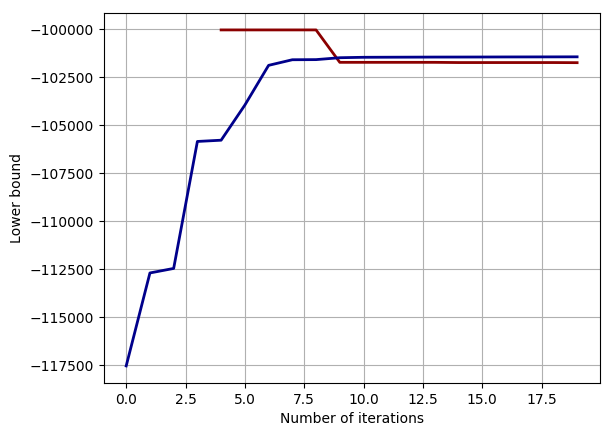In :
# and evolution of execution time:
plot(sddp.stats.exectime, lw=2, c="k")
grid()

xlabel("Number of iterations")
ylabel("Time (s)");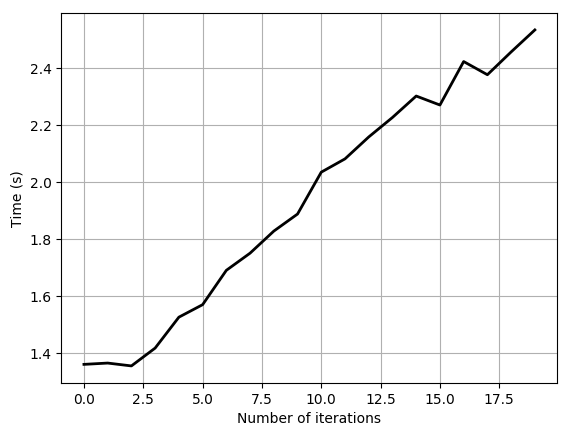The algorithm returns the bellman functions (V) and a vector of JuMP.Model used to approximate these functions with linear cuts.

Test SDDP with an example¶

Input scenario¶

We suppose given a scenario of inflows:

In :
alea_year = Array([7.0 7.0 8.0 3.0 1.0 1.0 3.0 4.0 3.0 2.0 6.0 5.0 2.0 6.0 4.0 7.0 3.0 4.0 1.0 1.0 6.0 2.0 2.0 8.0 3.0 7.0 3.0 1.0 4.0 2.0 4.0 1.0 3.0 2.0 8.0 1.0 5.0 5.0 2.0 1.0 6.0 7.0 5.0 1.0 7.0 7.0 7.0 4.0 3.0 2.0 8.0 7.0])
Out:
1×52 Array{Float64,2}:
7.0  7.0  8.0  3.0  1.0  1.0  3.0  4.0  …  7.0  7.0  4.0  3.0  2.0  8.0  7.0

We store this scenario as a 3D array, so it could be used to compute a forward-pass:

In :
aleas = zeros(52, 1, 1)
aleas[:, 1, 1] = alea_year;

SDDP simulation¶

We have only one scenario, so we set the forwardPassNumber equal to 1:

Find the optimal control with a forward simulation:

In :
costs, stocks = StochDynamicProgramming.simulate(sddp, 100);

Results¶

The cost is:

In :
SDDP_COST = costs
println("SDDP cost: ", SDDP_COST)
SDDP cost: -99495.47365243685

And the optimal solution is:

In :
plot(stocks[:, :, 1])
plot(stocks[:, :, 2])
xlabel("Week")
ylabel("Stocks")
grid()
ylim(0, 100)
xlim(0, 53);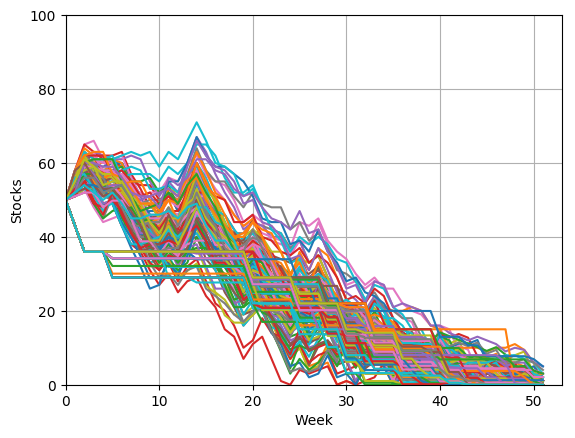Comparison with the deterministic solution¶

To check the results given by SDDP, we solve the deterministic problem with JuMP:

In :
m = Model(solver=solver)

@variable(m,  VOLUME_MIN  <= x1[1:(TF+1)]  <= VOLUME_MAX)
@variable(m,  VOLUME_MIN  <= x2[1:(TF+1)]  <= VOLUME_MAX)
@variable(m,  CONTROL_MIN <= u1[1:TF]  <= CONTROL_MAX)
@variable(m,  CONTROL_MIN <= u2[1:TF]  <= CONTROL_MAX)
@variable(m, u3[1:TF] >= 0)
@variable(m, u4[1:TF] >= 0)

@objective(m, Min, sum(COST[i]*(u1[i] + u2[i]) for i = 1:TF))

for i in 1:TF
@constraint(m, x1[i+1] - x1[i] + u1[i] + u3[i] - alea_year[i] == 0)
@constraint(m, x2[i+1] - x2[i] + u2[i] + u4[i] - u1[i] - u3[i] == 0)
end

@constraint(m, x1 == x0)
@constraint(m, x2 == x0)

status = solve(m)
println(status)

LP_COST = getobjectivevalue(m)
println("LP value: ", LP_COST)
Optimal
LP value: -104297.05688368101

And we plot the evolution of the stocks:

In :
plot(getvalue(x1))
plot(getvalue(x2))
xlabel("Week")
ylabel("Stocks")
grid()
ylim(0, 100)
xlim(0, 53);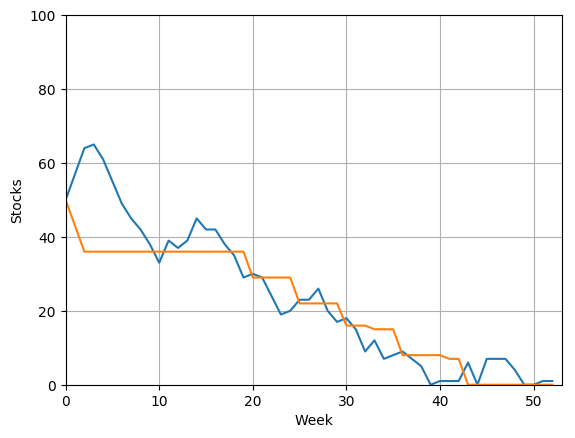The solution given by the solver is more optimistic, as it assumes that the future is known in advance.

If we consider the costs, we have a discrepancy between the two solutions :

In :
abs((LP_COST - SDDP_COST)/LP_COST)
Out:
0.046037571669919765